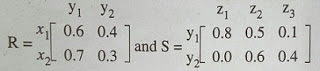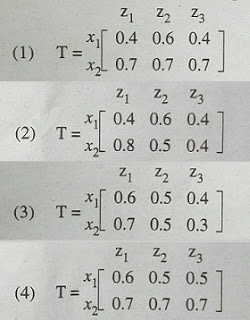1. Consider a fuzzy set A defined on the interval X = [0, 10] of integers by the membership Junction μA(x) = x / (x+2)Then the α cut corresponding to α = 0.5 will be a. {0, 1, 2, 3, 4, 5, 6, 7, 8, 9, 10} b. {1, 2, 3, 4, 5, 6, 7, 8, 9, 10} c. {2, 3, 4, 5, 6, 7, 8, 9, 10} d. None of the above
 Answer: (c).{2, 3, 4, 5, 6, 7, 8, 9, 10}

 2. If A and B are two fuzzy sets with membership functions:μa(χ) ={0.2,0.5.,0.6,0.1,0.9}μb (χ)= {0.1,0.5,0.2,0.7,0.8}then the value of μa ∩ μb  will be a. {0.2,0.5,0.6,0.7,0.9} b. {0.2, 0.5,0.2, 0.1,0.8} c. {0.1, 0.5, 0.6, 0.1,0.8} d. {0.1, 0.5, 0.2, 0.1,0.8}

 3. The height h(A) of a fuzzy set A is defined ash(A) = sup A(x) a. h(A) = 0 b. h(A) <0 c. h(A)=1 d. h(A)<1

 4. A __________ point of a fuzzy set A is a point x ∈ X at which µA(x) = 0.5 a. Core b. Support c. Cross-over d. α - cut

 5. Suppose the function y and a fuzzy integer number around -4 for x are given as y= (x-3)2 + 2.Around -4 = {(2, 0.3), (3, 0.6), (4, 1), (5, 0.6), (6, 0.3)} respectively. Then f (Around -4) is given by: a. {(2, 0.6), (3, 0.3), (6, 1), (11, 0.3)} b. {(2, 0.6), (3, 1), (6, 1), (11, 0.3)} c. {(2, 0.6), (3, 1), (6, 0.6), (11, 0.3)} d. {(2, 0.6), (3, 0.3), (6, 0.6), (11, 0.3)}
 Answer: (c).{(2, 0.6), (3, 1), (6, 0.6), (11, 0.3)}

 6. Given  U =  {1,2,3,4,5,6,7}              A = {(3, 0.7), (5, 1), (6, 0.8)} then A will be: (where ~ → complement) a. {(4, 0.7), (2,1), (1,0.8)} b. {(4, 0.3.): (5, 0), (6. 0.2) } c. {(l, 1), (2, 1), (3, 0.3), (4, 1), (6,0.2), (7, 1)} d. {(3, 0.3), (6.0.2)}
 Answer: (c).{(l, 1), (2, 1), (3, 0.3), (4, 1), (6,0.2), (7, 1)}

 7. Consider a fuzzy set old as defined below Old = {(20, 0.1), (30, 0.2), (40, 0.4), (50, 0.6), (60, 0.8), (70, 1), (80, 1)} Then the alpha-cut for alpha = 0.4 for the set old will be a. {(40,0.4)} b. {50, 60, 70, 80} c. {(20, 0.1), (30, 0.2)} d. {(20,  0),  (30,  0),  (40,   1), (50,1), (60, 1), (70, 1), (80, 1)}
 Answer: (d).{(20,  0),  (30,  0),  (40,   1), (50,1), (60, 1), (70, 1), (80, 1)}

 8. Perceptron learning, Delta learning and LMS learning are learning methods which falls under the category of a. Error correction learning  -  learning with a teacher b. Reinforcement learning - learning with a critic c. Hebbian learning d. Competitive learning - learning without a teacher
 Answer: (a).Error correction learning  -  learning with a teacher

 9. Let R and S be two fuzzy relations defined as follows. Then, the resulting relation, T, which relates elements of universe x to the elements of universe z using max-min composition is given by:a. (1) b. (2) c. (3) d. (4)